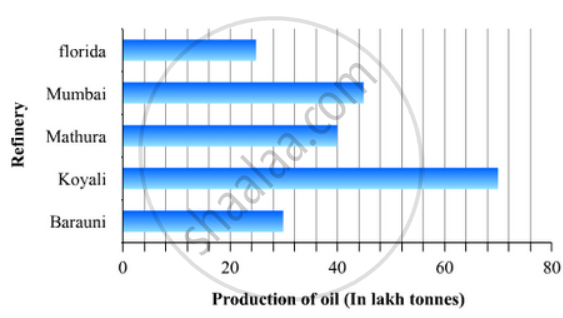# The Production of Oil (In Lakh Tonnes) in Some of the Refineries in India During 1982 Was Given Below: - Mathematics

The production of oil (in lakh tonnes) in some of the refineries in India during 1982 was given below:

 Refinery: Barauni Koyali Mathura Mumbai Florida Production of oil(in lakh tonnes) 30 70 40 45 25

Construct a bar graph to represent the above data so that the bars are drawn horizontally.

#### Solution

To represent the given data by a vertical bar graph, we first draw horizontal and vertical axes. Let us consider that the vertical and horizontal axes representing the refineries and the production of oil in lakh tonnes respectively. We have to draw 5 bars of different lengths given in the table.

At first we mark 5 points in the vertical axis at equal distances and erect rectangles of the same width at these points. The lengths of the rectangles are proportional to the productions of oil.

The horizontal bar graph of the given data is following:Concept: Graphical Representation of Data
Is there an error in this question or solution?

#### APPEARS IN

RD Sharma Mathematics for Class 9
Chapter 23 Graphical Representation of Statistical Data
Exercise 23.2 | Q 17 | Page 28

Share